GFG App
Open AppBrowser
Continue

# What is the Formula for Finding Angles?

In geometry, an angle is an essential measurement of a geometric shape. An angle is defined as the degree of rotation about the point of intersection between two lines or planes that are required to bring one into correspondence with the other. There are various kinds of angles, based on the measurement of an angle. It is measured in terms of degrees or radians. An angle is a shape formed by two lines or rays that diverge from a common point called a vertex. When two rays are intersected, i.e., when half-lines are projected with a common endpoint, an angle is formed. Now, the common endpoints are called vertices, while the rays are known as the arms.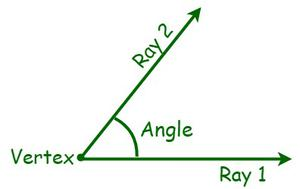### Types of Angles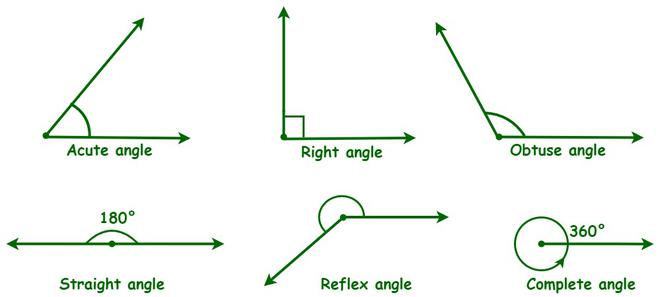1. Acute angle: An acute angle is an angle that is greater than 0 degrees and less than 90 degrees, i.e., it ranges from 0° to 90° (both exclusive).
2. Right angle: A right angle is referred to as the angle that measures exactly 90 degrees.
3. Obtuse angle: An obtuse angle is an angle that is greater than 90 degrees and less than 180 degrees, i.e., it ranges from 90° to 180°(both exclusive).
4. Straight angle: A straight angle is referred to as an angle that measures exactly 180 degrees.
5. Reflex angle: A reflex angle is an angle that is greater than 180 degrees and less than 360 degrees, i.e., it ranges from 180° to 360° (both exclusive).
6. A complete angle or Full Rotation: A complete angle is referred to as the angle that measures exactly 360 degrees.

There are also other types of angles, such as complementary angles, supplementary angles, and adjacent and non-adjacent angles.

• Complementary angles: Two angles are said to be complementary if their sum is a right angle, i.e., 90°.
• Supplementary angles: Two angles are said to be supplementary if their sum is equal to 180°.
• Adjacent angles: Two angles are said to be adjacent if they share a common vertex and a common arm.
• Non-adjacent angles: Two angles are said to be non-adjacent if they do not share a common vertex and a common arm.

### The formula for Finding Angles

There are various types of formulas for finding an angle; some of them are the central angle formula, double-angle formula, half-angle formula, compound angle formula, interior angle formula, etc.

• We use the central angle formula to determine the angle of a segment made in a circle.
• We use the sum of the interior angles formula to determine the missing angle in a polygon.
• We use the trigonometric ratios to find the missing angle of a right-angled triangle.
• We use the law of sines or the law of cosines to find the missing angle of a non-right angle triangle.

### Sample Questions

Question 1: Find the angle at the vertex B of the given triangle using one of the trigonometric formulae for finding angles.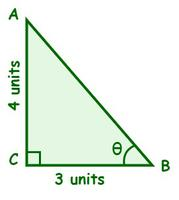Solution:

Given,

BC = 3 units = Adjacent side of θ.

AC = 4 units = Opposite side of θ.

In this case, we know both the opposite and adjacent sides of θ. Hence, we can use the tangent formula to find θ.

⇒ tan θ = opposite side/adjacent side

⇒ tan θ = 4/3

⇒ θ = tan-1 (4/3) ⇒ θ = 53.1°

Hence, the angle at vertex B is 53.1°.

Question 2: Find the angles at vertices X and Y, if ∠Z = 35° and x = 3 inches, y = 8 inches, and z = 3.5 inches.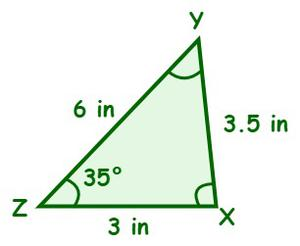Solution:

Given,

∠Z = 35° and x = 6 inches, y = 3 inches, and z = 3.5 inches

Since we know all three sides and an angle, we can use the sine rule formula.

From the sine rule formula, we have

x/sin X = y/ sin Y = z/sin Z

Now,

y/ sin Y = z/sin Z

⇒ 3/sin Y = 3.5/sin 35°

⇒ 3/sin Y = 3.5/0.574    {Since, sin 35° = 0.574}

⇒ sin Y = 3 × (0.574/3.5) = 0.492

⇒ ∠Y = sin−1(0.492) = 29.47°

We know that, sum of three angles in a triangle is 180°.

⇒ ∠X + ∠Y + ∠Z = 180°

⇒ ∠X + 29.47° + 35° = 180°

⇒ ∠X = 180° – 64.47‬° = 115.53°

Hence, ∠X = 115.53‬° and ∠Y = 29.47°.

Question 3: Calculate the fifth interior angle of a pentagon if four of its interior angles are 110°, 85°, 136°, and 105°.

Solution:

The number of sides of a pentagon (n) = 5.

Now, the sum of all 5 interior angles of a pentagon = 180 (n -2)°

= 180 (5 – 2)° = 540°.

The sum of the given 4 interior angles = 110°+ 85°+ 136°+ and 105°= 436‬°.

So, the fifth interior angle = 540° – 436‬° = 104‬°

Thus, the fifth interior angle of a pentagon is 104‬°.

Question 4: Determine the value of y and also the measure of angles in the given figure.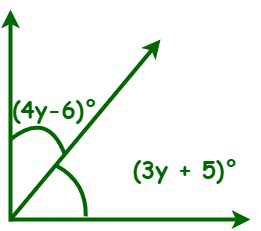Solution:

From the given figure, we can observe that (4y – 6)‬° and (3y + 5)‬° are complementary angles, i.e., the sum of (4y – 6)‬° and (3y + 5)‬° is 90‬°.

⇒ (4y – 6)‬° + (3y + 5)‬° = 90‬°

⇒ (7y – 1)‬° = 90‬°

⇒ 7y = 90‬° + 1‬° = 91‬°

⇒ y = 91‬°/7 = 13‬°

Now, (4y – 6)° = (4 ×13 – 6)° = (52 – 6)° = 46°

(3y + 5)° = (3 × 13 + 5)° = (39 + 5)° = 44°

Question 5: Find the angle at vertex Q in the given triangle using one of the formulas for finding angles.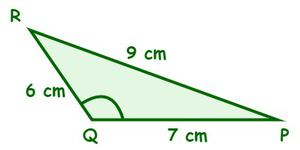Solution:

Given, p = QR = 6 cm,  q = PR = 9 cm, and  r = PQ = 7 cm.

Since we know all three sides and an angle, we can use the cosine rule formula to find the angle vertex Q.

⇒ q2 = p2 + r2 – 2pr cos Q

⇒ 92 = 62 + 72 – 2 (6)(7) cos Q

⇒ 81 = 36 + 49 – 84 cos Q

⇒ 81 = 85 – 84cos Q

⇒84 cos Q = 81 – 85

⇒ 84 cos Q = -4

⇒ cos Q = -4/84 = -1/21

⇒ ∠Q = cos-1(-1/21) = 92.72°

Hence, the angle at vertex Q, ∠Q = 92.72°.

Question 6: Calculate the angle of a segment made in a circle if the arc length is 12π and the radius is 9 cm.

Solution:

Given,

The arc length= 12π

Now, the angle formula is:

⇒ θ = (s×360°)/2πr

⇒ θ = (12π × 360°)/(2π × 5)

⇒ θ =12 ×360°/10

⇒ θ = 240°

Hence, the angle is 240°.

My Personal Notes arrow_drop_up